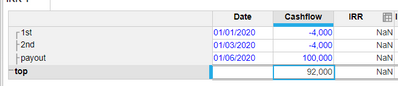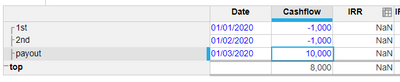disclaimer

## issue

IRR returns NaN when some of the following conditions are satisfied:

• There a small number of cashflows (less than 5)
• The difference between the numbers is large (>10,000)
• The resulting IRR value is large (over 500)

The current implementation will fail to calculate IRR when the above situations are satisfied and it will return NaN. The reason for this is because of the iterative nature of the function fails to converge the large void between the numbers.## workaround

1. If the values have a lot of significant figures, using ROUND on the cashflows can help as it changes the numbers. This will cause a slight loss of accuracy though and the rounding value will have to be adjusted on a case by case basis.
2. Create a read/write connection in the Anaplan Excel Add-in. Use Excels XIRR function to calculate the value and then write the data back to Anaplan.